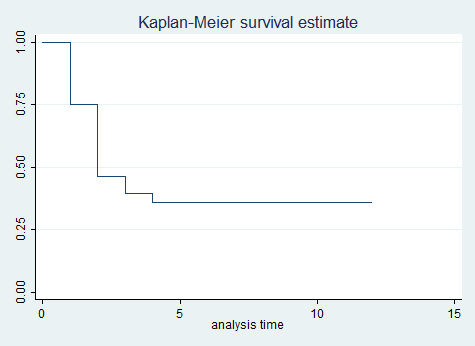»  Home »  Resources & support »  FAQs »  Missing values reported by stsum

## Why does stsum sometimes report missing values for the percentiles of survival time?

 Title Missing values reported by stsum Author Mario Cleves, StataCorp

Look at the following stsum output:

 . webuse stsum, clear

. stsum, by(exp)

failure _d:  disease
analysis time _t:  time

|               incidence       no. of    |------ Survival time -----|
exp      | time at risk     rate        subjects        25%       50%       75%
---------+---------------------------------------------------------------------
0 |           83   .2650602            29          1         2         5
1 |           93   .1935484            28          1         2         .
---------+---------------------------------------------------------------------
total |          176   .2272727            57          1         2         .


See the missing value for the 75th percentile of survival time for the group exp==1? That is the question: why is that value missing? In more extreme cases, you might see both the 50th and 75th percentile estimates, or even all three, missing.

In any case, the short answer is that the estimates are missing because the percentiles cannot be estimated. The percentiles of survival time reported are for completed survival times, and they are obtained from the Kaplan–Meier product-limit estimates of the survival curve. Let’s look at that curve for this group:

 . sts graph if exp==1The survivor function S(t) reports the probability of surviving at least to time t. The survivor function here becomes flat at S(t) = .333. It does that because not all the subjects have died yet—this is called right-censoring.

What is the 25th percentile of completed survival times? The 25th percentile occurs where S(t) = 1−.25 =.75 (meaning that 25% have failed and 75% have yet to fail), and that is t=1. Look back at the stsum output and you will see that 1 is reported.

What is the 50th percentile of completed survival times? The 50th percentile occurs where S(t) = 1−.50 = .50, and that is t=2. stsum reports that number, too.

What is the 75th percentile? We do not know because more than 25% of the subjects have not failed yet. Thus stsum reports the 75th percentile for survival time as missing.